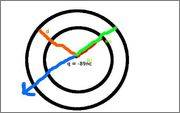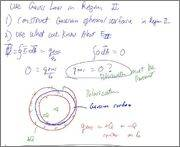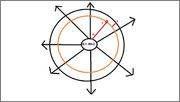# Trouble with Gauss Law

• blackbyron

## Homework Statement

A hollow conducting sphere carries a charge of 72nC and has an inner radius of 44cm and an outer radius of 68cm. At the center of the hollow sphere is a point charge (-89)nC, insulated from the sphere. What is the magnitude of the electric field inside the sphere at a distance of 9.5cm from the inner surface?

## Homework Equations

E = Q*r/(4*pi*Eo*R^3)

## The Attempt at a Solution

I don't find my teacher notes helpful.
I've been googling on Gauss Law and i know about the concepts. The problem is I am lost on where to start off the problem. I just don't understand what to do. Anyhelp will be appreicated.

Thanks.

How would you apply Gauss's law here? Where would you put your Gaussian surface? What does Gauss's law state?

Thanks for your reply. Well, I'm using it to find out the electric field.

I know that rb > r > ra which makes this one zero.

There are two charges, so where does the charge 72nC locate at?
Is this one a correct drawing?E = Qk/r^2

E = Qk/r^2

That's Coulomb's Law. What does Gauss' Law state?

Oh, I'm wondering why my professor uses the columb's law.

Gauss states that phi = Q/Eo, is that right, according to my professors notes.

Oh, I'm wondering why my professor uses the columb's law.
Well, you could use Coulomb's law, but let's stick to Gauss's law.
Gauss states that phi = Q/Eo, is that right, according to my professors notes.
Yes. What's phi and how would you calculate it? Again, where would you put the Gaussian surface? What's its radius?

Oh, I'm wondering why my professor uses the columb's law.
We'll get to that bit shortlyGauss states that phi = Q/Eo, is that right, according to my professors notes.

Okay, what specifically does the Q represent in that formula? What, in words, does Gauss' Law state?

phi is a net electric field.

When I look at this video http://www.youtube.com/watch?v=QNIJC1emss8&feature=related

Q represents the sum of the charges? Is that right?

I put phi inside the Gaussian surface, not sure if that answer to question.

For calculations on phi, the thing that concerns is the constant Eo. It's unknown, and puts me into problems. The thing that my teacher show in the notes is to do flux integration show that it's coulombs law.

phi is a net electric field.
Not quite, it's the total electric flux passing through the Gaussian surface. It's not the value of the electric field itself (which is a vector E, not $\Phi$).
Q represents the sum of the charges? Is that right?
Ah! But which charges? Where must these charges be located?
I put phi inside the Gaussian surface, not sure if that answer to question.

For calculations on phi, the thing that concerns is the constant Eo. It's unknown, and puts me into problems. The thing that my teacher show in the notes is to do flux integration show that it's coulombs law.
Eo (or more correctly, $\epsilon_0$) is the known physical constant representing the permittivity of empty space.

The thing that you want to recognize from Gauss' law is that the net sum of the flux through the Gaussian surface depends ONLY on charges located WITHIN that closed surface. This should also tell you something about the net electric field that occurs inside of a uniform spherically distributed charge (like the charge on the outer conducting sphere), and hence the influence that that outside charge will have on any field inside.

Now, where are you planning to locate a Gaussian surface to address the question posed in your problem? What charge(s) will be inside of it? What charge(s) will be outside of it?

Now, where are you planning to locate a Gaussian surface to address the question posed in your problem? What charge(s) will be inside of it? What charge(s) will be outside of it?

Hi,

The -89nC which is a point charge goes to the center and the 72nC goes to the inner radius. Is that right?
I'm using my teacher notes like thisOkay. That diagram depicts a case where the Gaussian surface is placed within the body of the conductive shell. Where do you want your Gaussian surface for the problem you're trying to solve?

Okay. That diagram depicts a case where the Gaussian surface is placed within the body of the conductive shell. Where do you want your Gaussian surface for the problem you're trying to solve?

Wait, does that matter? Because all I do is follow what my instructor tells me. Maybe i'll just put the same position that my instructor did on the notes. I don't think I understand the use of Gaussian surface. I'm sorry if I'm wasting you time answering my questions.

Wait, does that matter? Because all I do is follow what my instructor tells me. Maybe i'll just put the same position that my instructor did on the notes. I don't think I understand the use of Gaussian surface. I'm sorry if I'm wasting you time answering my questions.

No problem. The location matters because you're trying to determine something about the electric field at a particular location. You want to determine which charges will be affecting the field there.

I am going to say that the electric field is located in the inner radius.Where is the location of the point that you want to find the field? Is it between the inner charge and the shell, or within the shell, or outside of the shell?

Where is the location of the point that you want to find the field? Is it between the inner charge and the shell, or within the shell, or outside of the shell?

EDIT:
I think I got it. The location of the electric field will be inside the inner shell near the point charge.

Now the Electric field of the charge 72 is zero because ro > r > ra.

Is that right?

Then I use a point charge to find the electric field. Not sure I'm making sense because my communication is not that good.

Last edited:
EDIT:
I think I got it. The location of the electric field will be inside the inner shell near the point charge.

Now the Electric field of the charge 72 is zero because ro > r > ra.

Is that right?

Then I use a point charge to find the electric field. Not sure I'm making sense because my communication is not that good.

You're doing fine.

So, the location of interest is between the central point charge and the shell. Gauss' Law then tells you that the charge on the shell will have no effect on the field inside that shell, so the only charge that matters is the central one. Time for Coulomb's law!Now I'm getting it. Just find out the net Electric Field and there I go. Thanks. I appreciate your help :)

sorry not "ro > r >ra" meant to say "ro > r > ri"